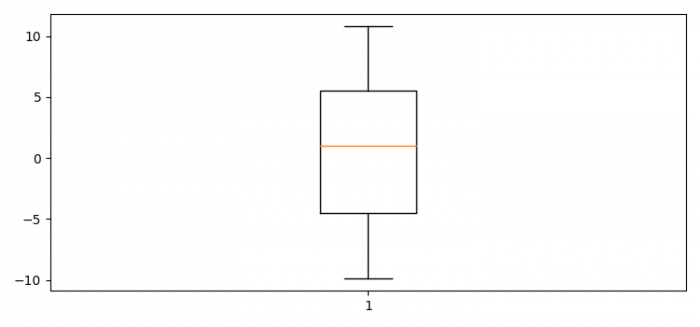# How to deal with NaN values while plotting a boxplot using Python Matplotlib?

To deal with NaN value while plotting a boxplot using Python, we can take the following steps −

## Steps

• Set the figure size and adjust the padding between and around the subplots.

• Initialize a variable N for data samples and for range.

• Next create the random spread, center's data, flier high and low, get the concatenated data, and the filtered data.

• Create a box plot using boxplot() method.

• To display the figure, use show() method.

## Example

import matplotlib.pyplot as plt
import numpy as np

# Set the figure size
plt.rcParams["figure.figsize"] = [7.50, 3.50]
plt.rcParams["figure.autolayout"] = True

# Data samples
N = 10

# Center's data
center = np.ones(N)

# Flier high and low
fh = np.random.rand(N)+N
fl = np.random.rand(N)-N

# Concatenated data
data = np.concatenate((spread, center, fh, fl), 0)
data = np.NaN

# Filtered data
filtered_data = data[~np.isnan(data)]

# Plot the boxplot
plt.boxplot(filtered_data)

plt.show()

## Output

It will produce the following output −